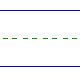Name: ______________________________________________ Date: _____________

Multiplication

Instructions: Multiply.

 12 x 4 =12 x 11 =12 x 2 =12 x 0 =9 x 12 =7 x 12 =4 x 12 =12 x 8 =8 x 12 =11 x 12 =12 x 10 =0 x 12 =6 x 12 =12 x 1 =10 x 12 =12 x 12 =3 x 12 =12 x 3 =